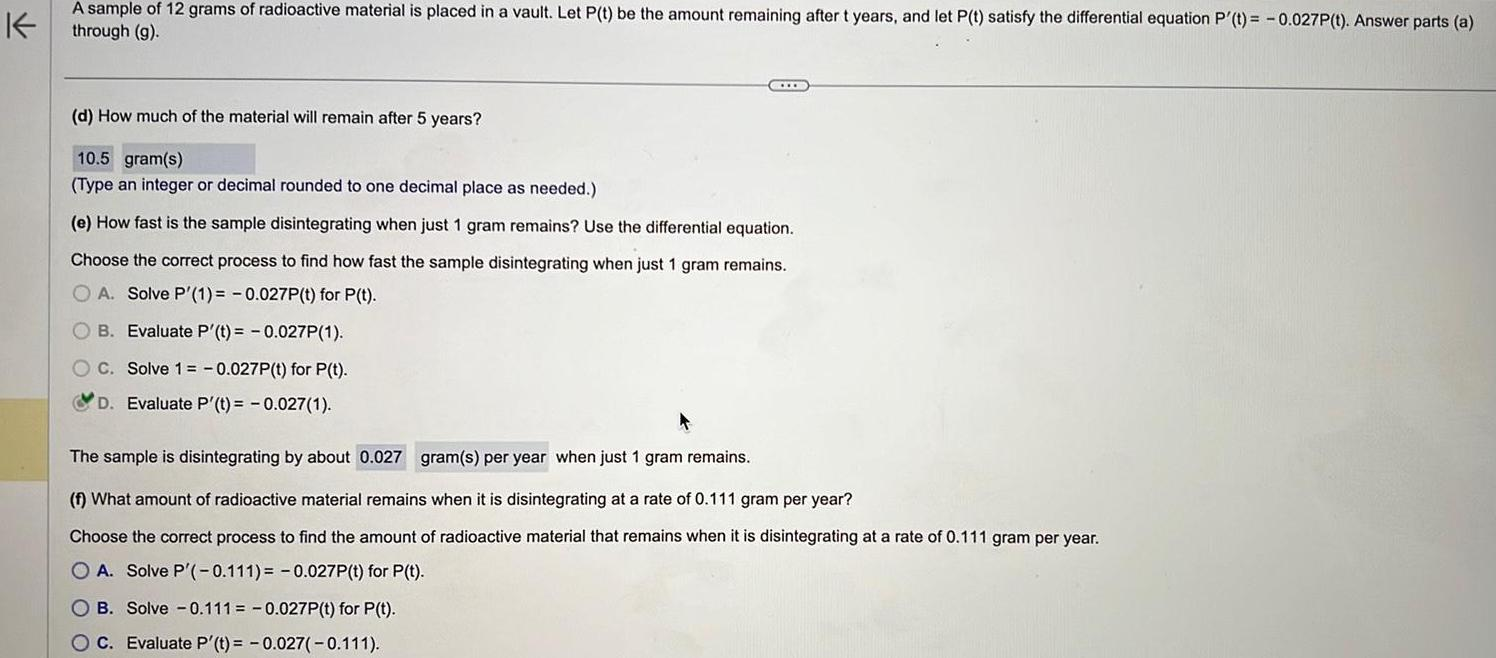Question:

# K A sample of 12 grams of radioactive material is placed in

Last updated: 9/19/2023K A sample of 12 grams of radioactive material is placed in a vault Let P t be the amount remaining after t years and let P t satisfy the differential equation P t 0 027P t Answer parts a through g d How much of the material will remain after 5 years 10 5 gram s Type an integer or decimal rounded to one decimal place as needed e How fast is the sample disintegrating when just 1 gram remains Use the differential equation Choose the correct process to find how fast the sample disintegrating when just 1 gram remains OA Solve P 1 0 027P t for P t B Evaluate P t 0 027P 1 C Solve 1 0 027P t for P t D Evaluate P t 0 027 1 The sample is disintegrating by about 0 027 gram s per year when just 1 gram remains f What amount of radioactive material remains when it is disintegrating at a rate of 0 111 gram per year Choose the correct process to find the amount of radioactive material that remains when it is disintegrating at a rate of 0 111 gram per year OA Solve P 0 111 0 027P t for P t OB Solve 0 111 0 027P t for P t C Evaluate P t 0 027 0 111